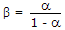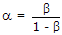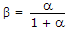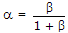# Electronics and Communication Engineering - Power Electronics

### Exercise :: Power Electronics - Section 8

16.

In a single phase half wave ac regulator feeding a resistive load, the rms load current

 A. is always positive B. may be positive or negative C. is always negative D. can be even zero

Explanation:

No answer description available for this question. Let us discuss.

17.

Which of the following is correct for a BJT?

 A.B.C.D.Explanation:

No answer description available for this question. Let us discuss.

18.

In a bridge inverter with single pulse width modulation

 A. the output frequency is equal to frequency of reference signal B. the output frequency is equal to frequency of control signal C. either (a) or (b) D. neither (a) nor (b)

Explanation:

No answer description available for this question. Let us discuss.

19.

In a modified series inverter with two inductors and two capacitors, the load current

 A. has 2 parallel paths B. has only one parallel path C. can have three parallel paths D. has four parallel paths

Explanation:

No answer description available for this question. Let us discuss.

20.

The number of layers in a Shockley diode are

 A. 2 B. 3 C. 4 D. 2 or 3